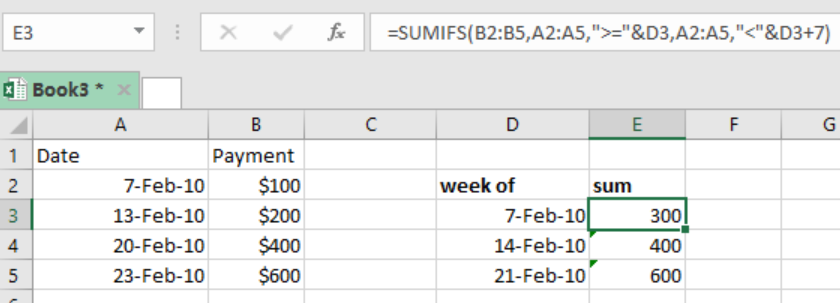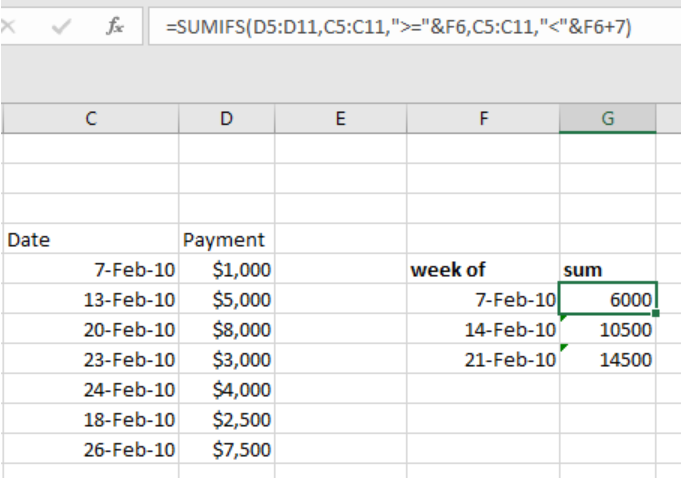Get instant live expert help with Excel or Google Sheets“My Excelchat expert helped me in less than 20 minutes, saving me what would have been 5 hours of work!”

#### Post your problem and you'll get expert help in seconds

Your message must be at least 40 characters
Our professional experts are available now. Your privacy is guaranteed.

# How to Calculate the Sum by Week in Excel

We can now use a formula based on the excel SUMIFS function to sum by week. This article provides a clear guide on how you can use the function to sum by week.Figure 1: How to sum by week

## General syntax of the formula

`=SUMIFS(value, date, “>=”&A1, date, “<”&A1+7)`

Where;

Value- refers to what you want to sum

Date- refers to the dates of the week for which you want to sum

## Understanding the formula

The SUMIFS function plays a critical role when it comes to summing values by week.

In our example above, we have found the sum of the payments by week.

To do this, all we need to do is specify the formula and it will help us sum by week

## How the formula works

• We can use the SUMIFS function to sum ranges based on multiple criteria
• In our case here, we want the SUMIFS function to sum amounts based on two criteria which are;
1. dates that are equal or greater than date in column D.
2. dates that are equal to or less than those in column D plus 7 days
• After we have found the sum for the first column, we need to copy down the formula to the other cells to get the sums for the other entries.

## ExampleFigure 2: Example of how to sum by week

In this example, we want to find the sum of all the payments. To do this, proceed as follows;

Step 1: fill the table with the data

Step 2: Indicate where you want the results to appear, in our case, the results should appear in column G.

Step 3: In cell G6, put the formula; `=SUMIFS(D5:D11,C5:C11,">="&F6,C5:C11,"<"&F6+7)`

Step 4: Press Enter to get the sum based on that week

Step 5: Copy down the formula to the other cells and get the sum based on their respective weeks

## Instant Connection to an Expert through our Excelchat Service

Most of the time, the problem you will need to solve will be more complex than a simple application of a formula or function. If you want to save hours of research and frustration, try our live Excelchat service! Our Excel Experts are available 24/7 to answer any Excel question you may have. We guarantee a connection within 30 seconds and a customized solution within 20 minutes.

### Did this post not answer your question? Get a solution from connecting with the expert.Another blog reader asked this question today on Excelchat:## Subscribe to Excelchat.coAnother blog reader asked this question today on Excelchat: